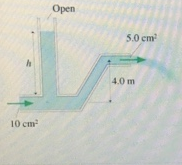# Problem: Water flows from the pipe shown in the figure with a speed of 8.0 m/s. (Figure 1)What is the height h of the standing column of water? Express your answer to two significant figures and include the appropriate units.

###### FREE Expert Solution

Continuity equation:

$\overline{){\mathbf{Av}}{\mathbf{=}}{\mathbit{c}}{\mathbit{o}}{\mathbit{n}}{\mathbit{s}}{\mathbf{tan}}{\mathbit{t}}}$

Bernoulli’s equation:

$\overline{){{\mathbf{P}}}_{{\mathbf{1}}}{\mathbf{+}}\frac{\mathbf{1}}{\mathbf{2}}{\mathbf{\rho }}{{{\mathbf{v}}}_{{\mathbf{1}}}}^{{\mathbf{2}}}{\mathbf{+}}{\mathbf{\rho }}{\mathbf{g}}{{\mathbf{y}}}_{{\mathbf{1}}}{\mathbf{=}}{{\mathbf{P}}}_{{\mathbf{2}}}{\mathbf{+}}\frac{\mathbf{1}}{\mathbf{2}}{\mathbf{\rho }}{{{\mathbf{v}}}_{{\mathbf{2}}}}^{{\mathbf{2}}}{\mathbf{+}}{\mathbf{\rho }}{\mathbf{g}}{{\mathbf{y}}}_{{\mathbf{2}}}}$

We'll use 1 to identify the inlet and 2 to identify the outlet.

A1 = 10 cm2

A2 = 5 cm2

v2 = 8.0 m/s

A1v1 = A2v2

v1 = (A2v2)/A1 = (5 ×  8.0)/10 = 4.0 m/s

Using the inlet as the reference point:

89% (41 ratings)###### Problem Details

Water flows from the pipe shown in the figure with a speed of 8.0 m/s. (Figure 1)What is the height h of the standing column of water? Express your answer to two significant figures and include the appropriate units.